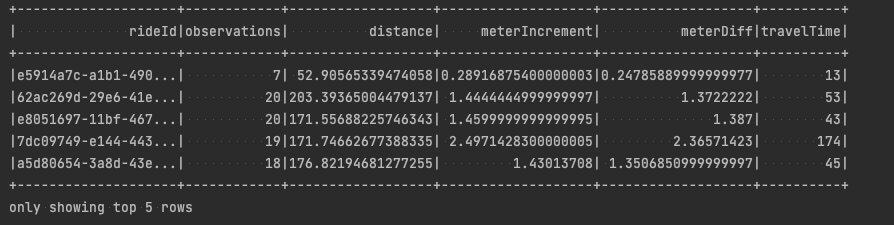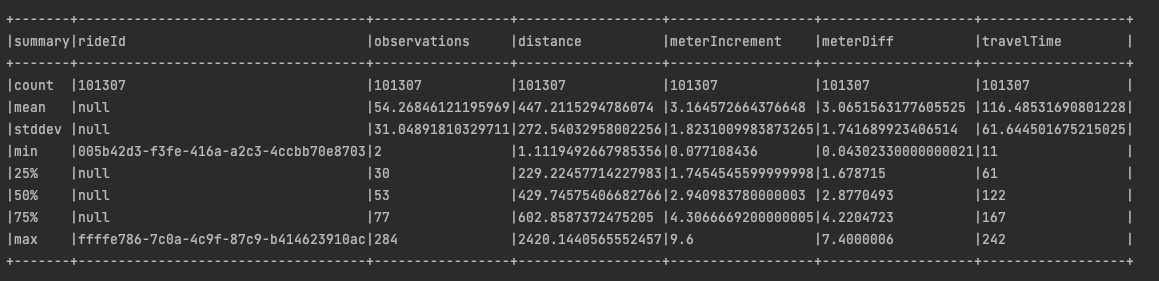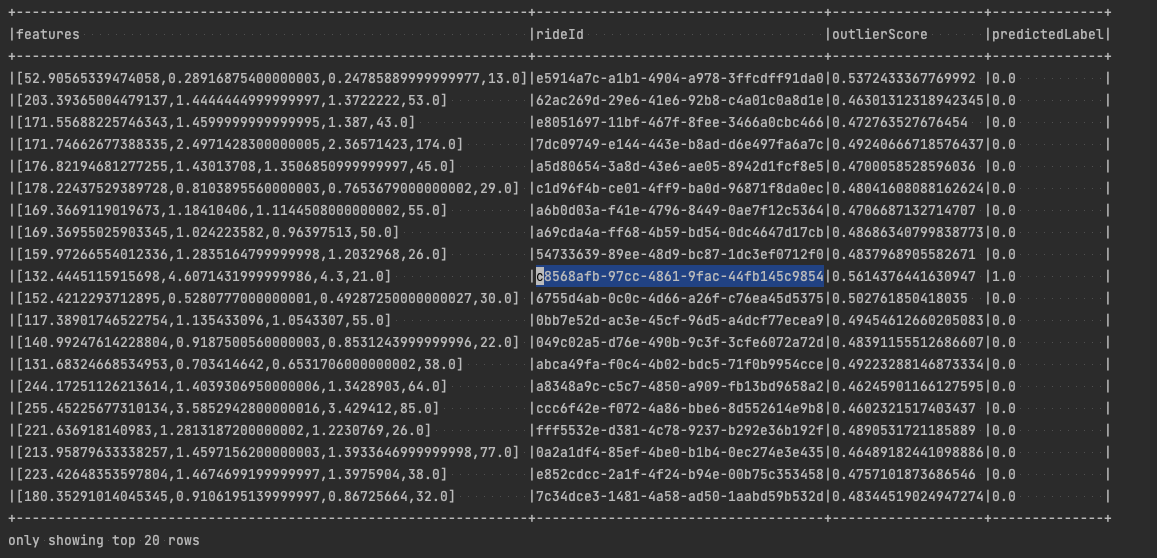# Isolation Forest Anomaly Detection with Spark and NYC Taxi Data Stream

In my previous post, I wrote about how to use Spark with a custom GCP PubSub subscription receiver. Today, I will try to develop an anomaly detection solution, incorporating the aforementioned receiver and data from the New York City Tycoon Taxi data stream.

We will divide our work into 4 stages where we prepare the training data, train our ML model, check for anomalies, and evaluate the results.#### Tiomax 80 @Flickr

First, we need to gather enough data so we can train our Spark ML model. To do this, we will use our custom PubSub receiver we have implemented before, process the data a bit during the stream processing phase, and store the stream after processing with the classic Spark `saveAsTextFiles(..)` function.

## Preparing the training data

The first part of this section is about setting up the Spark Context and utilising our `PubSubCustomReceiver` to do its work.

``````Logger.getLogger("org").setLevel(Level.ERROR)

val spark = SparkSession
.builder
.appName("StructuredStreaming")
.master("local[*]")
.getOrCreate()

val ssc = new StreamingContext(spark.sparkContext, Seconds(1))
ssc.checkpoint("data/checkpoint/")

"data/service-key.json"))
val messages = messagesRaw.map(v => parseTR(v))``````

The `parseTr()` method was described in the previous post, and it is all about parsing the received message into a case class:

``````def parseTR(str: String): TaxiRide = {
implicit val taxiRideFormat: OFormat[TaxiRide] = Json.format[TaxiRide]
Json.parse(str).as[TaxiRide]
}

case class TaxiRide (
ride_id: String,
point_idx: Long,
latitude: Double,
longitude: Double,
timestamp: LocalDateTime,
meter_increment: Double,
ride_status: String,
passenger_count: Int)

case class Location(lat: Double, lon: Double)``````

Please note, however, that we now operate on the `LocalDateTime` type for the timestamp instead of a simple string, it turns out that the `Json` parser from `Play` can handle the strings in NYC Tycoon stream out of the box. We need the real temporal type here for the duration of the travel recorded within a single window, to calculate travel time in seconds for that entry (more on that below).

Once we have a stream of `TaxiRide` objects in hand, we can start processing it. We will basically convert the stream of a `TaxiRide's` into a stream of grouped taxi rides by `ride_id` and then convert the grouped taxi rides into a single row for each group.

``````val fullRides = messages.map(tr => (tr.ride_id, List(tr)))
.reduceByKeyAndWindow( (first, second) => first ++ second, (first, second) => second, Seconds(30), Seconds(10))
.filter { e =>
if(e._2.size >= 2) {
}else{
false
}
}
.map { e =>
val list = e._2.sortBy(_.ride_id)
(e._1, list.size,
calculateDistance(list),
calculateMeterIncrementSum(list),
calculateTravelTimeInSeconds(list)
)
}``````

In other words, we group taxi rides received in the original stream by `ride_id`, then we take all the rides with the same `ride_id` captured within 30 seconds, and calculate (every 10 seconds) the following properties:

• the number of observations captured — how many elements were in a single list grouped by ride_id
• the distance between the first and last points recorded using their latitude and longitude coordinates with the Haversines formula (in meters).
• the sum of all taxi meter increments
• the difference between the last and the first taxi meter reading

(the first point recorded is the one with the smallest `point_idx` and the last one with the greatest `point_idx`)

The reason we do the initial filtering on the grouped data is to make sure that the list of taxi rides we want to process contains the `ride_idx` as a list of consecutive numbers, otherwise we could end up with ids from the beginning of the ride and some from the end with missing values in between, distorting our results.

Side note: If you want to calculate the stats or anomalies for the whole ride, you would need to gather and process all the data available on the PubSub stream, which is not possible without setting up a proper computing cluster with quite a bit of computing power (The NYC Taxi Tycoon public Pub/Sub topic generates 2000 to 2500 simulated taxi ride updates per second — up to 8 Mb or more per second).

The distance is measured with a small utility method and the Haversine formula for getting the rough distance in meters between two locations on the globe. Meter readings calculations and travel times are self explanatory.

``````def calculateDistance(lst: List[TaxiRide]): Double = {
val AVERAGE_RADIUS_OF_EARTH_M = 6371000 //in meters
def calculateDistanceInMeters(locStart: Location, locEnd: Location): Double = {
val latDistance = Math.toRadians(locStart.lat - locEnd.lat)
val lngDistance = Math.toRadians(locStart.lon - locEnd.lon)
val sinLat = Math.sin(latDistance / 2)
val sinLng = Math.sin(lngDistance / 2)
val a = sinLat * sinLat +
sinLng * sinLng)
val c = 2 * Math.atan2(Math.sqrt(a), Math.sqrt(1 - a))
}
val minPoint = lst.minBy(_.point_idx)
val maxPoint = lst.maxBy(_.point_idx)
calculateDistanceInMeters(Location(minPoint.latitude, minPoint.longitude), Location(maxPoint.latitude, maxPoint.longitude))
}

def calculateMeterIncrementSum(lst: List[TaxiRide]): Double = {
lst.foldLeft(0.0)(_ + _.meter_increment)
}

def calculateMeterReadingDiff(lst: List[TaxiRide]): Double = {
val minPoint = lst.minBy(_.point_idx)
val maxPoint = lst.maxBy(_.point_idx)
}

def calculateTravelTimeInSeconds(lst: List[TaxiRide]): Long = {
val minPoint = lst.minBy(_.point_idx)
val maxPoint = lst.maxBy(_.point_idx)
ChronoUnit.SECONDS.between(minPoint.timestamp, maxPoint.timestamp)
}``````

Once we group the rides from a single sliding window in the stream and calculate the new properties, we store them in a file, so we can later build an ML model out of it.

``````val sortedResults = fullRides.transform(rdd => rdd.sortBy(x => x._2, ascending = false))
sortedResults.map(e => s"\${e._1},\${e._2},\${e._3},\${e._4},\${e._5},\${e._6}").saveAsTextFiles("data/taxi-output/taxi-rides-processed")

ssc.start()
ssc.awaitTermination()
// Stop the session
spark.stop()``````

For convenience, once you stop collecting data, you can navigate to the `data/taxi-output` directory and execute:

``find . -name "part-*" -exec cat {} + > ~/path-to-your-project/data/final.csv``

It will concatenate all the `part-*` files into a single csv result.

## Building the Isolation Forest model

When we have our data ready, we can start training our Isolation Forest model. We will use a library called `Spark-iForest` available on GitHub.

Isolation forests are pretty good for anomaly detection, and the library is easy to use and well described:

Isolation Forest (iForest) is an effective model that focuses on anomaly isolation. iForest uses tree structure for modeling data, iTree isolates anomalies closer to the root of the tree as compared to normal points. A anomaly score is calculated by iForest model to measure the abnormality of the data instances. The higher, the more abnormal.

But most importantly, Isolation Forest is an algorithm from the unsupervised learning category and we don’t need to have any data annotated upfront to start playing around with it.

First, we need to parse our data from the csv file into a `DataFrame`:

``````Logger.getLogger("org").setLevel(Level.ERROR)

val spark = SparkSession
.builder
.appName("Isolation Forest")
.master("local[*]")
.getOrCreate()

val taxiRideSchema = new StructType()

.format("csv")
.option("comment", "#")
.schema(taxiRideSchema)

After gathering about 100MB of data, and analysing it initially, I have found out that we have some records with negative values in taxi meter readings. Let’s fix that with simple filtering:

``````    val rawData = preRawData.filter(col("meterIncrement") > 0 && col("meterDiff") > 0 && col("travelTime") > 10)
rawData.show(5)``````

You can print the first couple of records after successfully loading the data into a `DataFrame`, just to check if everything works:Let’s print a summary for all the columns, so we can analyse the data further:

``    rawData.summary().show(20, false)``To use our data with the Isolation Forest, we need to first create a feature vector with all the columns we want to be included for finding anomalies. I have picked the `distance`, `meterIncrement`, `meterDiff`, and `travelTime`. This way, we can find anomalies for data like extreme cost of travel compared to distance and time passed.

``````val assembler = new VectorAssembler()
.setInputCols(Array("distance","meterIncrement","meterDiff","travelTime"))
.setOutputCol("features")
val data = assembler
.transform(rawData)
.select(col("features"), col("rideId"))

/**
* Train the model
*/

val contamination = 0.1
val isolationForest = new IsolationForest()
.setNumEstimators(100)
.setBootstrap(false)
.setMaxSamples(256)
.setMaxFeatures(1.0)
.setFeaturesCol("features")
.setPredictionCol("predictedLabel")
.setScoreCol("outlierScore")
.setContamination(contamination)
.setContaminationError(0.01 * contamination)
.setRandomSeed(1)

val isolationForestModel = isolationForest.fit(data)``````

The `isolationForest.fit(data)` function trains our model. We can store it and use it later with a batch or stream to detect anomalies in other unseen events from the NYC Tycoon Taxi data.

Parameter values used are sensible defaults for the Isolation Forest algorithm:

• maxSamples: The number of samples to draw from data to train each tree (>0). If maxSamples <= 1, the algorithm will draw maxSamples totalSample samples. If maxSamples > 1, the algorithm will draw maxSamples samples. The total memory is about maxSamples numTrees 4 + maxSamples 8 bytes. Note: The normFactor will be re-adjusted in the transform (predicton) function according to the size of the predicting dataset if maxSamples <= 1, which means the data instance with the same features may have different predictive scores for different size of dataset. Set a fixed maxSamples (maxSamples > 1), if you want a constant prediction scores in the transforming phase.
• maxFeatures: The number of features to draw from data to train each tree (>0). If maxFeatures <= 1, the algorithm will draw maxFeatures * totalFeatures features. If maxFeatures > 1, the algorithm will draw maxFeatures features.
• contamination: The proportion of outliers in the data set, the value should be in (0, 1). It is only used in the prediction phase to convert anomaly score to predicted labels. In order to enhance performance, Our method to get anomaly score threshold is caculated by approxQuantile. You can set the param approxQuantileRelativeError greater than 0, in order to calculate an approximate quantile threshold of anomaly scores for large dataset.
• bootstrap: If true, individual trees are fit on random subsets of the training data sampled with replacement. If false, sampling without replacement is performed.
• featuresCol: features column name, default “features”.
• predictionCol: Prediction column name, default “prediction”.

## Evaluating the results

We haven’t split our data into training and test as we didn’t have any labels associated with our records in the first place nor any other metrics available to evaluate our model. We can try to detect anomalies on the same data we used for training and see if they make any sense.

Let’s run and evaluate our prediction with a model created earlier in our tutorial and our data:

``````    val dataWithScores = isolationForestModel.transform(data)
dataWithScores.show(20, false)``````

The first 20 results get printed, we have one anomaly there with id `c8568afb-97cc-4861–9fac-44fb145c9854`When we check this record in our csv file, we see that it has the following values:

ride_idobservationsdistancemeterIncrementmeterDifftravelTime
c8568afb-97cc-4861-9fac-44fb145c985415132.44451159156984.60714319999999864.321

This can be translated to:

• price per km: 4.61 / (132.44 / 1000) = 34.81 USD
• price per hour: (3600 * 4.61) / 21 = 790.29 USD

The mean prices (from our summary printed earlier):

• price per km: 3.16 / (447.21 / 1000) = 7.06 USD
• price per hour: (3600 * 3.16) / 116.5 = 97.64 USD

Please note that the prices calculated above were using the data from an approximately 2-minute-long window only. The ride identified as an anomaly had data from only 21 seconds.

As you can see, our detection algorithm found a pretty bizarre data point where the same time spent in a taxi was over four times more expensive per km than the average!

Looking for more quality tech content about Machine Learning, Big Data or Stream Processing? Join Data Times, a monthly dose of tech news curated by SoftwareMill's engineers.